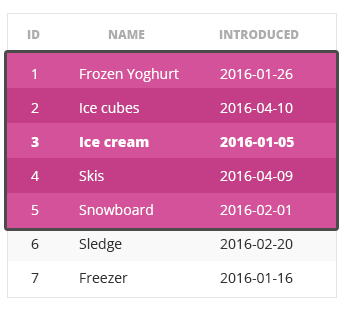Deals Of The Week - hours only!Up to 80% off on all courses and bundles.-Close
Introduction
ROWS
5. General syntax
RANGE
Default window frame
Summary

## Instruction

Alright. Let's get down to work. In this part, we're finally going to learn about window frames.

Window frames define precisely which rows should be taken into account when computing the results and are always relative to the current row. In this way, we can create new kinds of queries.

For instance, you may say that for each row, 3 rows before and 3 rows after it are taken into account; or rows from the beginning of the partition until the current row. In a moment, you'll discover how such queries can come in handy. Take a look at the example window frame, where two rows before and two rows after the current row are selected:The are two kinds of window frames: those with the keyword ROWS and those with RANGE instead. The general syntax is as follows:

<window function> OVER (...
ORDER BY <order_column>
[ROWS|RANGE] <window frame extent>
)


Of course, other elements might be added above (for instance, a PARTITION BY clause), which is why we put dots (...) in the brackets. For now, we'll focus on the meaning of ROWS and RANGE. We'll talk about PARTITION BY later in the course.

Let's take a look at the example:

SELECT
id,
total_price,
SUM(total_price) OVER(
ORDER BY placed
ROWS UNBOUNDED PRECEDING)
FROM single_order


In the above query, we sum the column total_price. For each row, we add the current row AND all the previously introduced rows (UNBOUNDED PRECEDING) to the sum. As a result, the sum will increase with each new order.

## Exercise

Click to run the template.